Next: Worked example 9.2: Angular Up: Angular momentum Previous: Angular momentum of a

## Worked example 9.1: Angular momentum of a missile

Question: A missile of mass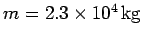flies level to the ground at an altitude of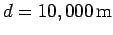with constant speed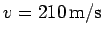. What is the magnitude of the missile's angular momentum relative to a point on the ground directly below its flight path?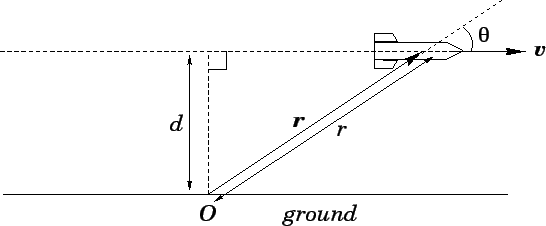Answer: The missile's angular momentum about pointis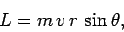whereis the angle subtended between the missile's velocity vector and its position vector relative to. However,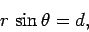whereis the distance of closest approach of the missile to point. Hence,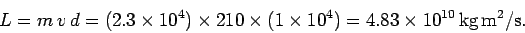Richard Fitzpatrick 2006-02-02